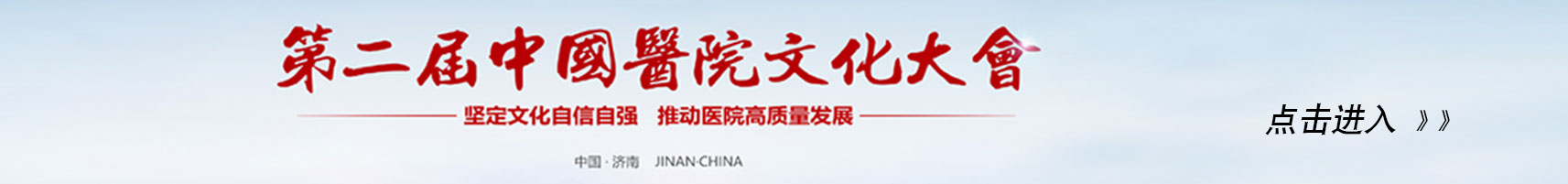## 欢迎进入山东省医院协会网站！ function showLocale(objD) { var str,colorhead,colorfoot; var yy = objD.getFullYear(); if(yy<1900) yy = yy+1900; var MM = objD.getMonth()+1; if(MM<10) MM = '0' + MM; var dd = objD.getDate(); if(dd<10) dd = '0' + dd; var hh = objD.getHours(); if(hh<10) hh = '0' + hh; var mm = objD.getMinutes(); if(mm<10) mm = '0' + mm; var ss = objD.getSeconds(); if(ss<10) ss = '0' + ss; var ww = objD.getDay(); if ( ww==0 ) colorhead="<font color=\"#FFF\">"; if ( ww > 0 && ww < 6 ) colorhead="<font color=\"#ffffff\">"; if ( ww==6 ) colorhead="<font color=\"#FFF\">"; if (ww==0) ww="星期日"; if (ww==1) ww="星期一"; if (ww==2) ww="星期二"; if (ww==3) ww="星期三"; if (ww==4) ww="星期四"; if (ww==5) ww="星期五"; if (ww==6) ww="星期六"; colorfoot="</font>" str = colorhead + yy + "-" + MM + "-" + dd + " " + hh + ":" + mm + ":" + ss + " " + ww + colorfoot; return(str); } function tick() { var today; today = new Date(); document.getElementById("localtime").innerHTML = showLocale(today); window.setTimeout("tick()", 1000); } tick();更多 》

更多 》

更多 》

## 出版图书

更多 》